# My Notes: Data Structures (CS50)

### 4/28/2020

To recap last week, some of the new concepts we learned about memory...

``````int main(void)
{
int *x;
int *y;

x = malloc(sizeof(int));

*x = 42;
*y = 13;
}
``````

What is the above code doing?

• Declaring `x` as a pointer
• Declaring `y` as a pointer
• Allocating space in memory to hold value of `x`
• Assign 42 to the memory that was assigned to `x`
• ! Assign 13 to `y` but there is no memory allocated to `y`

### Arrays

A data structure which can hold multiple values, as before we were just working with integers, characters, etc.

We have to declare the size of arrays up front (static data type).

If we declare `[1, 2, 3]` and we want to add another value, `4`, can we?

Memory may look something like this already: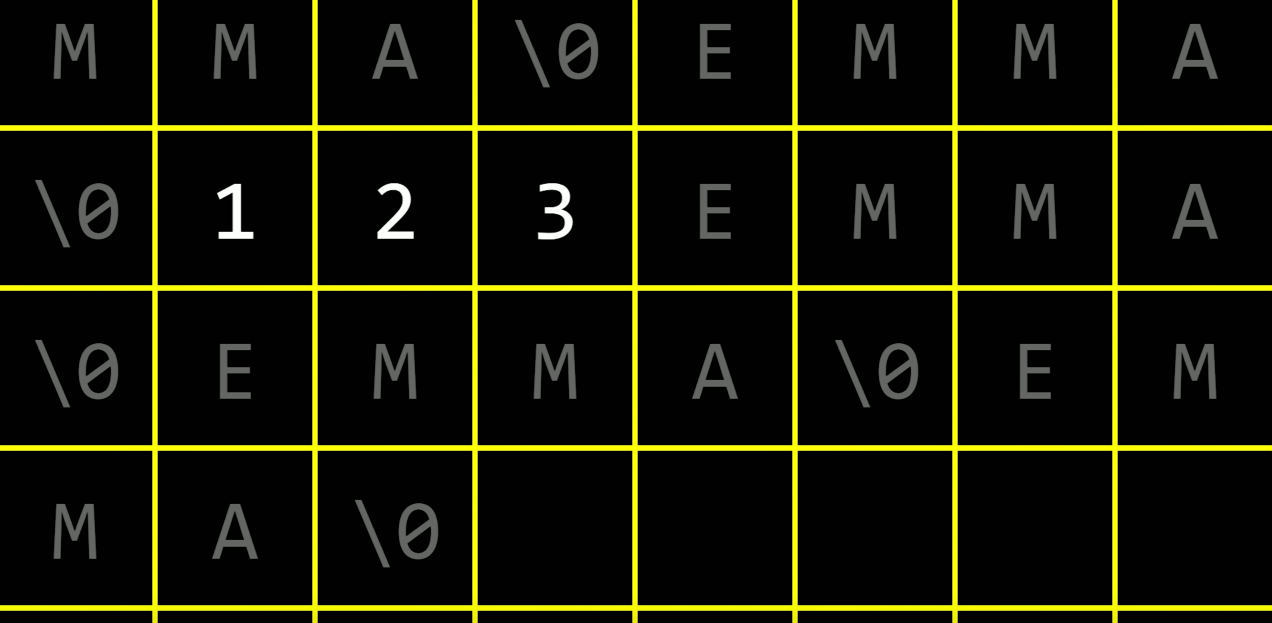Based on the above picture, it looks like there is still some available memory (empty boxes). We can maybe relocate the 1, 2, 3 to other memory space where there is space in the next box for 4.

We have to move/copy the new array to new memory space then we can throw away/free the old array. Is this the best way?

It takes up some time and effort to copy and move the array, it doesn't seem too efficient.

The running time is O(n) to insert a new value into the array. We had 3 values already in the array. So we have go through the array to reach the end and insert the value of 4. Let's see how this would look like in code.

``````int main(void)
{
int list;

list = 1;
list = 2;
list = 3;

for (int i = 0; i < 3; i++)
{
printf("%i\n", list[i]);
}

}
``````

Here we are hard coding an array with the size of 3, declaring the values, looping through the array, and printing out each value.

How can we change this to add a fourth value? Let's work on this code.

An array is a chunk of memory, pointers are an address of memory.

``````int main(void)
{
int *list = malloc(3 * sizeof(int));
if (list == NULL) // to check if we're out of memory
{
return 1;
}

list = 1;
list = 2;
list = 3;

for (int i = 0; i < 3; i++)
{
printf("%i\n", list[i]);
}

}
``````

Here we are setting a pointer, not using an array. So it is slightly less static code now. Below is how we can address possibly adding a fourth value.

``````int main(void)
{
int *list = malloc(3 * sizeof(int));
if (list == NULL) // to check if we're out of memory
{
return 1;
}

list = 1;
list = 2;
list = 3;

// Create memory for the new array
int *tmp = malloc(4 * sizeof(int));
if (tmp == NULL)
{
return 1;
}

// Copy list into tmp now
for (int i = 0; i < 3; i++)
{
tmp[i] = list[i];
}

// Set fourth value
tmp = 4;

// Free up memory from first array
free(list);

// Re-name tmp to list
list = tmp;

// Print new array
for (int i = 0; i < 4; i++)
{
printf("%i\n", list[i]);
}

}
``````

In the above code, we used `malloc()` to declare a new temporary pointer with more space, copy `list` values into the new `tmp` pointer with a for loop, then add the fourth value into `tmp`. We then free up the original array's memory, using `free(list)`, re-declare list to equal tmp, and print the values.

Another quicker, shorter way to do this is to use `realloc()`. Instead of creating a new temporary pointer, we can reallocate the memory and increase the size. Then we can shorten our code, we do not have to use a for loop to copy.

``````int main(void)
{
int *list = malloc(3 * sizeof(int));
if (list == NULL)
{
return 1;
}

list = 1;
list = 2;
list = 3;

int *tmp = realloc(list, 4 * sizeof(int));
if (tmp == NULL)
{
return 1;
}

list = tmp;

tmp = 4;

for (int i = 0; i < 4; i++)
{
printf("%i\n", list[i]);
}

free(list);

}
``````

If we try to just reassign `list` with `malloc()` instead of making a `tmp` pointer, it will work but we will lose the pointer to the original memory space, so it will still exist but we will not be able to retrieve it.

## Data Structures

A programming construct that allow you to store information differently in your computer's memory.

In C, there are three pieces of syntax which contribute to data structures -

• `struct` : keyword that allows you create your own structure (data type that contains multiple data types, creating a Person last week)
• `.` : to access the property of a structure
• `*` : de-reference operator to go to a chunk of memory through a pointer

An array is a fixed chunk as memory, if we want to add values, we need to do the above ^^ Inserting into them may not be very performant. Benefits of an array is that they are indexed, so they can be super fast when searching.

A linked list is a data structure that contains multiple chunks of memory, that are somehow linked together (by pointers here).

Linked lists don't have to be stored continously next to each other like an array but each list item will take two places of memory. One is to hold the value, the next piece is to hold a pointer to the next value in the list.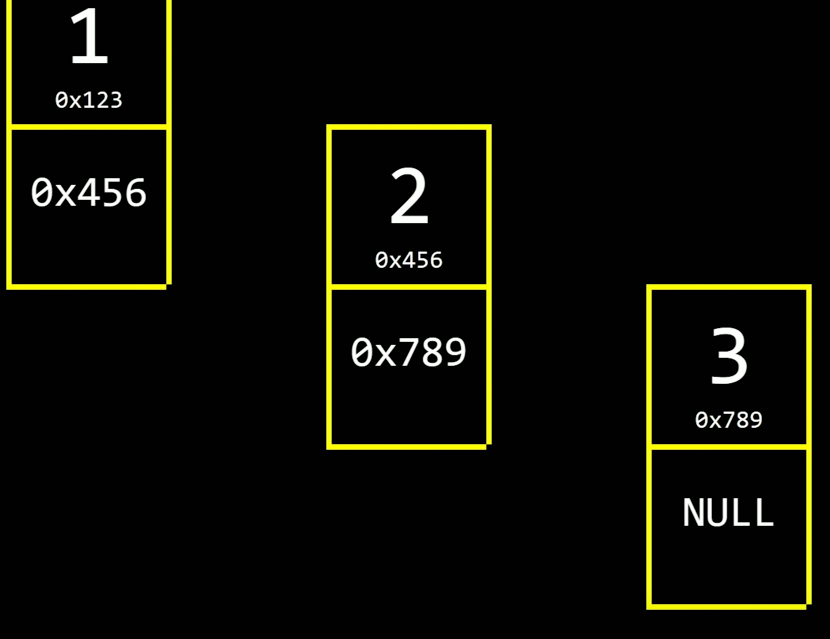Two fields: one integer, one next (pointer)

``````typedef struct node
{
int number;
struct node *next;
}
node;
``````

A linked list is made up of nodes, a data type defined above. Each node has a number and a pointer to a node structure.

Here is how to start a linked list, now that the typedef node has been created above.

``````node *n = malloc(sizeof(node));
if (n != NULL)
{
n->number = 2;
n->next = NULL;
}
``````

We create a node and assign the number 2 as its value. We declare next as NULL since there is no other node in the list yet.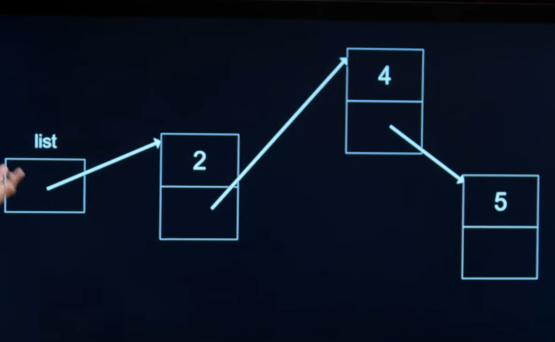One thing we lose from using linked lists (instead of arrays) is random access. Since arrays are continuous and indexed, we can't just access the middle of the index. With linked lists, we need to follow the nodes and traverse to go to a specific point.

Binary search is most efficient with random access.

Search and insert are O(n). Need to go through the whole list.

Here is a code implementation of a linked list:

``````#include <stdio.h>
#include <stdlib.h>

// Represents a node
typedef struct node
{
int number;
struct node *next;
}
node;

int main(void)
{
// List size 0
node *list = NULL;

node *n = malloc(sizeof(node));
if (n == NULL)
{
return 1;
}
n->number = 1;
n->next = NULL;
list = n;

// Add number (2) to list
n = malloc(sizeof(node));
if (n == NULL)
{
return 1;
}
n->number = 2;
n->next = NULL;
list->next = n;

// Add number (3) to list
n = malloc(sizeof(node));
if (n == NULL)
{
return 1;
}
n->number = 3;
n->next = NULL;
list->next->next = n;

// Print list
for (node *tmp = list; tmp != NULL; tmp = tmp->next)
{
printf("%i\n", tmp->number);
}

// Free list
while (list != NULL)
{
node *tmo = list->next;
free(list);
list = tmp;
}
}
``````

### Trees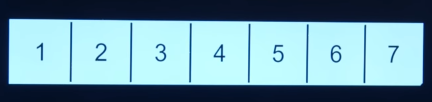We had worked with this array earlier, and done a binary search on it. We start in middle here, then go left or right, and continue to go into middles and split it.

Here is how we picture it in two dimensions.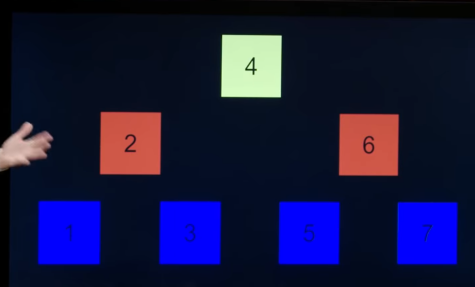It kind of has a tree shape. We still start in middle (4), then go left or right. It looks like it can be a linked list but we don't want to go in a sorted order (1-7).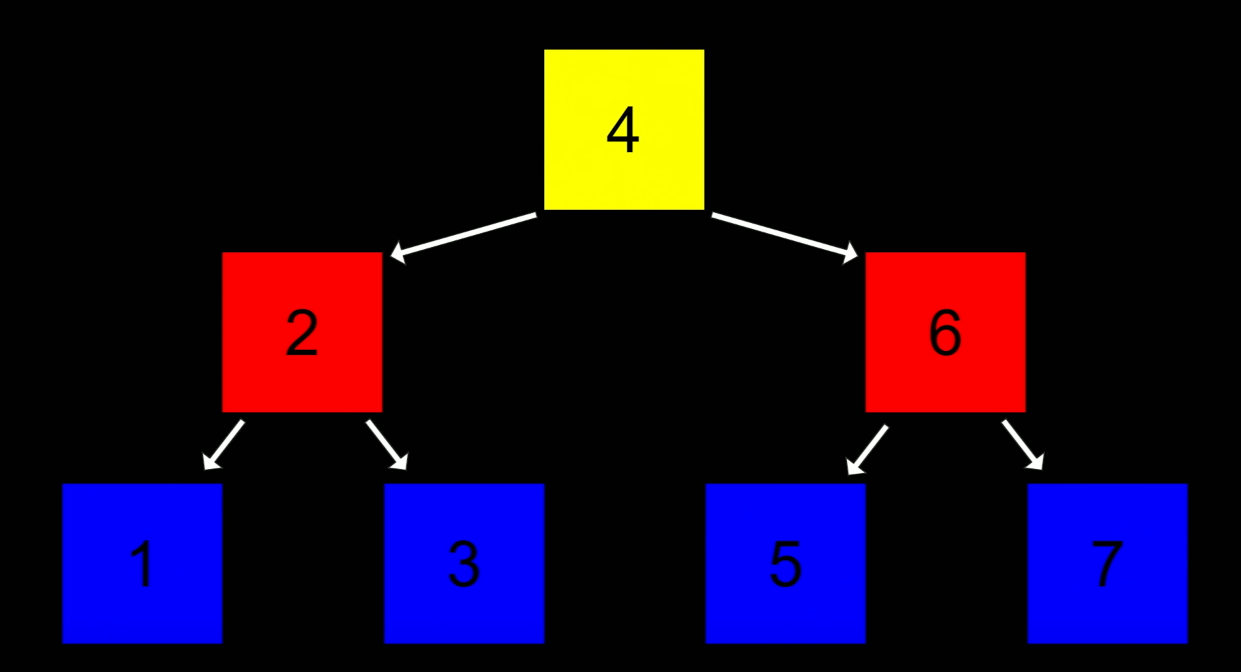We can construct nodes with two pointers (instead of a next, but a left and right).

``````typedef struct node
{
int number;
struct node *left;
struct node *right;
}
node;
``````

The above nodes can be used to implement the above tree, a Binary Search Tree (BST).

For any node in the tree, every element to the left is smaller, every element to the right is larger. It is recursive in the sense that every node follows that order (smaller to left, larger to right).

We're still using pointers, more dynamic, and we have our binary search back.

The root of the BST is on top, the `4` in the above tree.

Just like searching a list, we just need the beginning node. When you are searching a tree, you just need the root node to begin. Trees are recursive since they are just made up of trees.

``````bool search(node *tree)
{
if (tree == NULL)
{
return false;
}
else if (50 < tree->number)
{
return search(tree->left);
}
else if (50 > tree->number)
{
return search(tree->right);
}
else if (50 == tree->number)
{
return true;
}
}
``````

Binary Search Tree, search = O(log n)

Binary Search Tree, insert = O(log n)

### Hash Tables

A combination of an array and a linked list inside of it.

For example, if we're holding nametags at an event. We want to distribute them efficiently. If we have a register table, and they are sorted A-Z, but in one big pile, attendees will still have to go through all the name tags.

If we organize it by putting buckets, from A-Z, on the table. If you're name is Bob, you can go right for the B bucket.

For the buckets, we can use an array. If we're inserting names in an array with 26 indexes (for each alphabet), what happens when Bob is filled in and Beau needs to be inserted into the bucket? That is where hash tables come in. Our array will contain linked lists for each alphabet.

`[{A}, {B}, {C}, ..., {Z}]`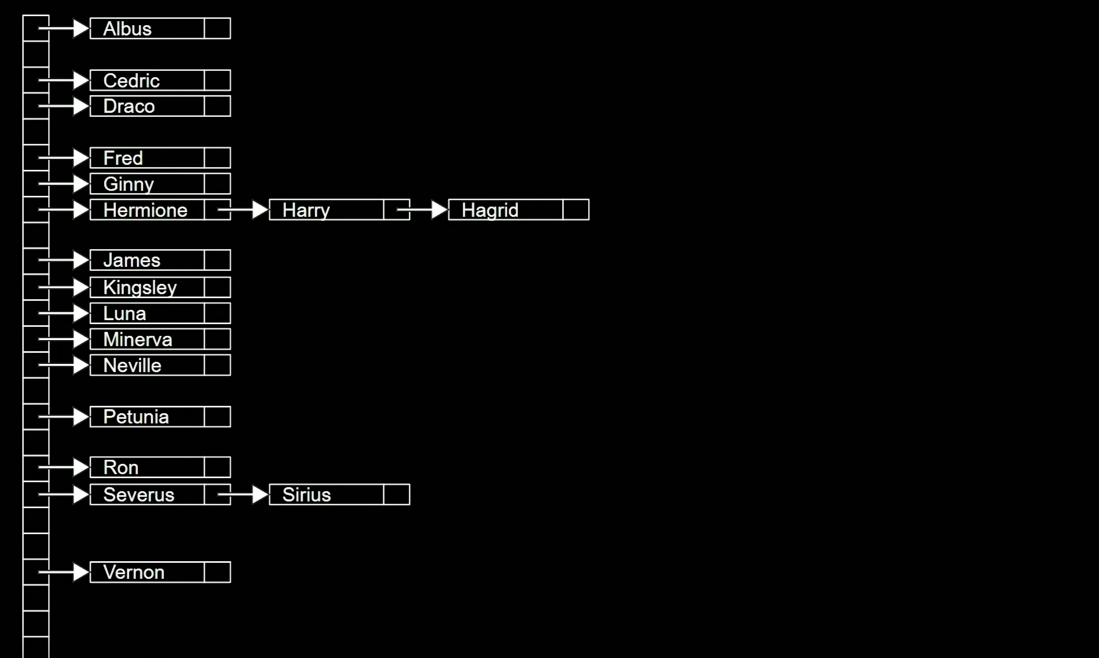We use a hash function to determine where our input will be stored in our hash bucket. For this nametag activity, we just search the first letter of each name. We will run into collisions here. The worst case for searching and inserting is O(n). We might just have that chance where there are many A's in the class.

How to mitigate this?

Maybe look at the first two letters? Might reduce collisions. There will still be collisions. There are more buckets but collisions seem unavoidable. You must determine the time and space tradeoff.

We must find the ideal hash function. In most cases, hash table search is O(n).

### Tries

Another data structure. Short for retrieval. Follows a pattern to use one resource to save on another (either time or space). A tree, each node being an array.

If you want to store a name in a trie, you look at every character in the name. If there is no child node, allocate another child node. Draw another array.

Here is trying to insert Hagrid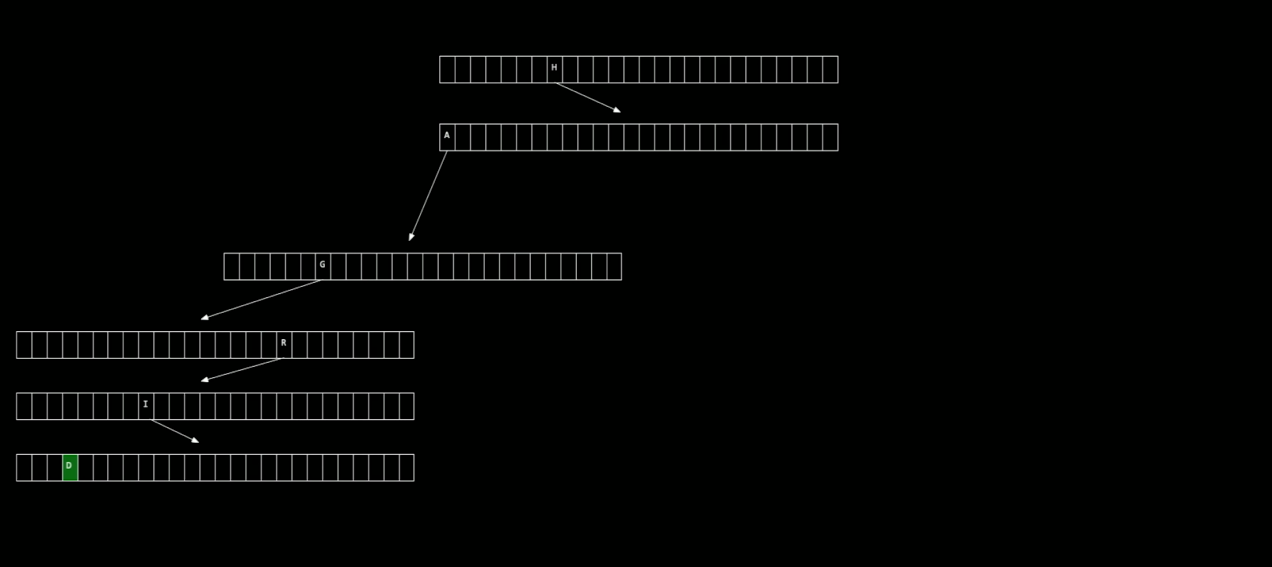Storing one node in every letter in the name.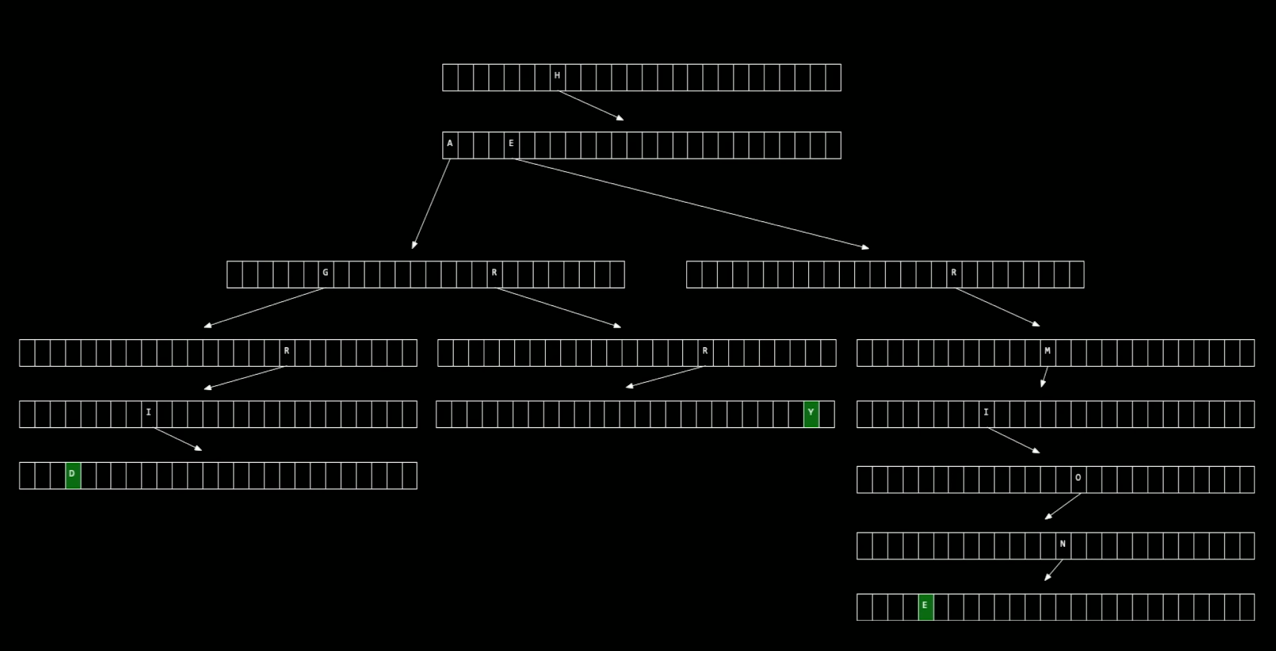Here we are inserting Hagrid, Harry, and Hermoine.

Some of the nodes are being shared. If there are n people in the tries, what is the running time to search? How many steps does it take? Count the number of characters in the name. Effectively, it is constant time, O(1), to search or insert.

Our running time is now low to search, but we're really using a lot of memory.

### Abstract Data Structures

We've been introduced to new data structures (arrays, linked lists, trees, hash tables), so we can try to solve some of the early problems we've faced.

#### Queues

FIFO Data structure. First in, first out. Think of a line at a grocery store. If you print paper in a college computer station, the printer system is a queue. It is printed in the order it was requested.

In queues, there are two operations:

• enqueue (get into the line)
• dequeue (finish and leave the line)

We can implement queues with an array or a linked list.

#### Stacks

Opposite principle of queues, stacks are LIFO, last in first out. Like dining trays at a dining hall. You take the tray from the top of the stack, not from the bottom. If you use email, the most recent messages go on top.

Operations in stacks:

• push (add to the stack)
• pop (remove from the stack)

#### Dictionaries

Abstraction on top of the hash bucket. A data structure that has keys and values. An English dictionary, has a word and its definition, a key-value pair.